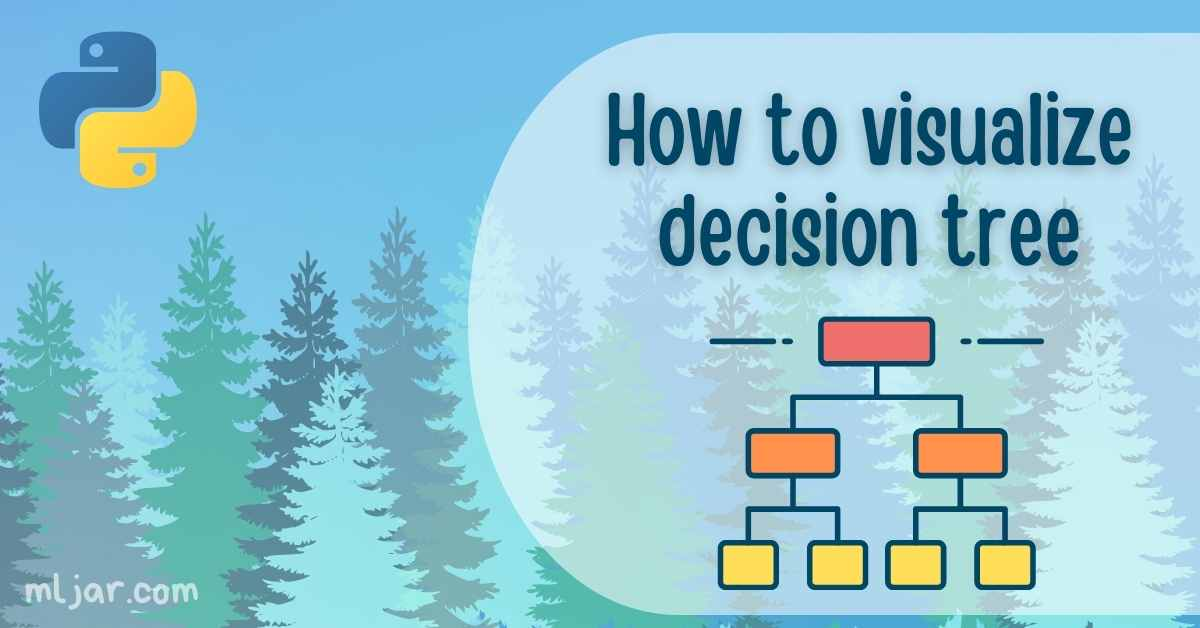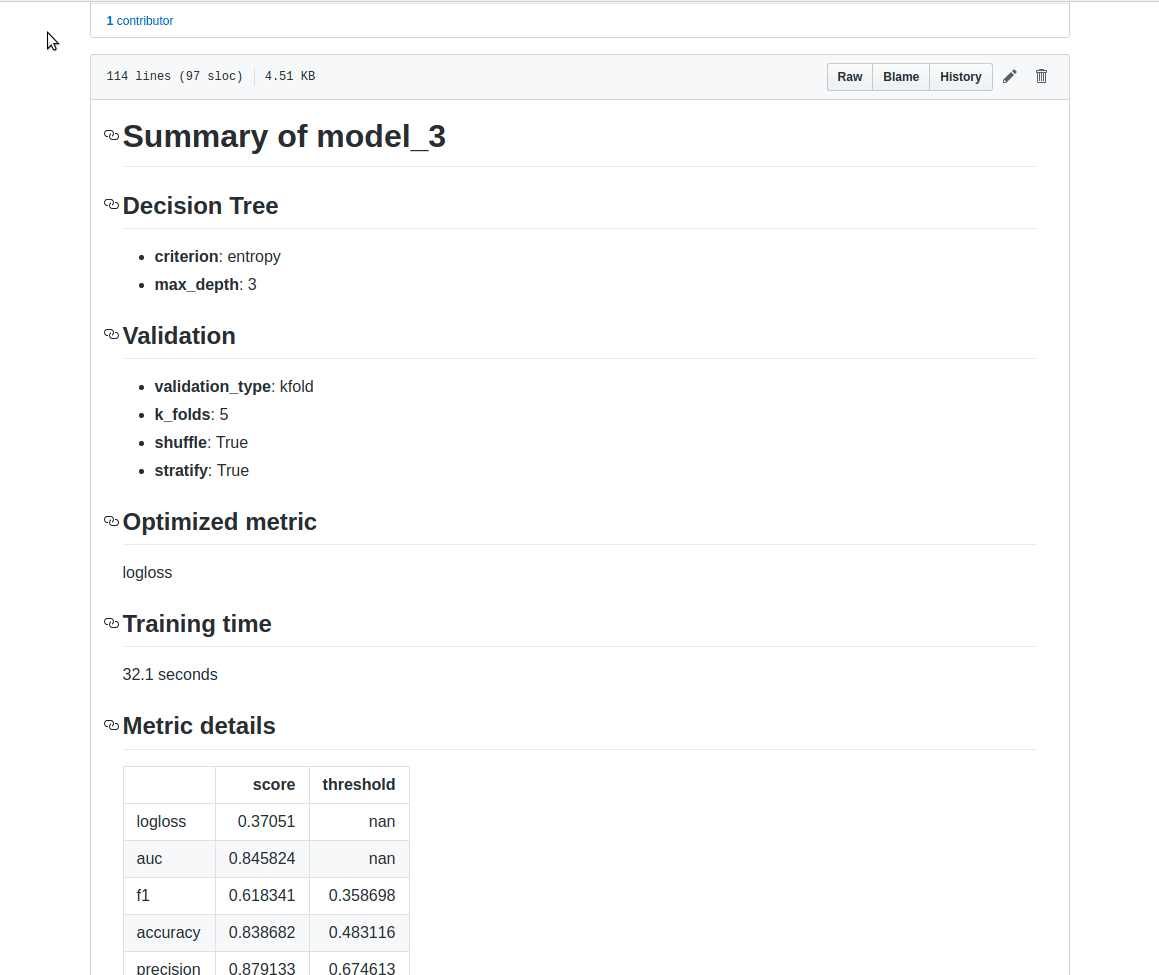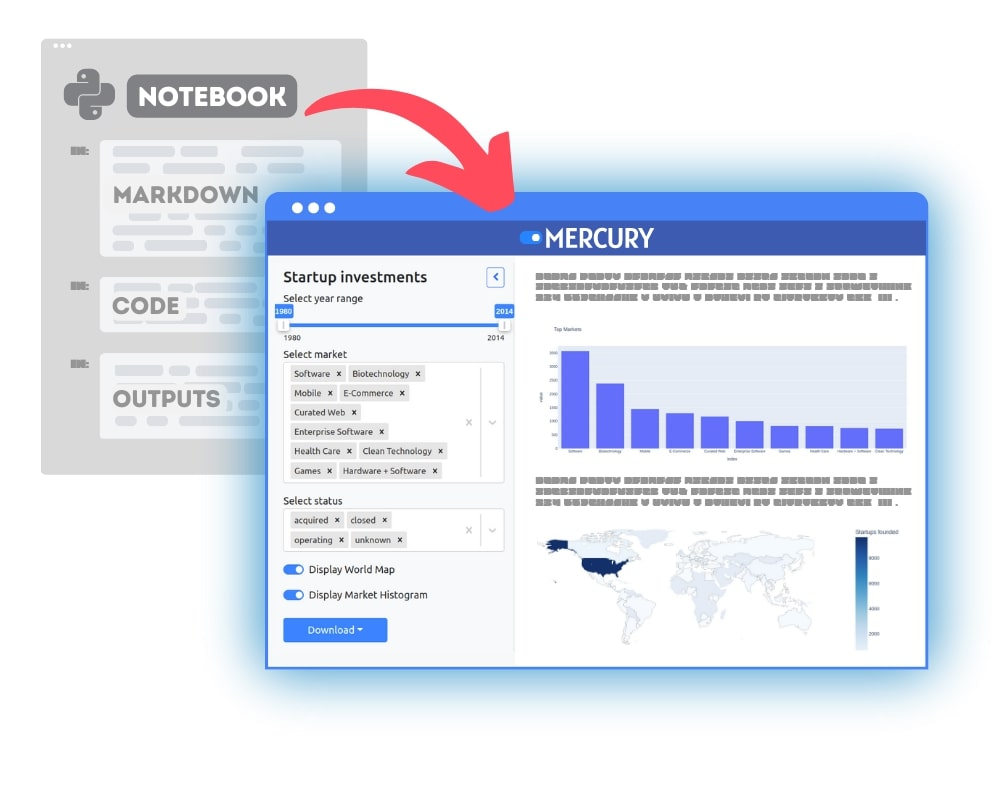A Decision Tree is a supervised algorithm used in machine learning. It is using a binary tree graph (each node has two children) to assign for each data sample a target value. The target values are presented in the tree leaves. To reach to the leaf, the sample is propagated through nodes, starting at the root node. In each node a decision is made, to which descendant node it should go. A decision is made based on the selected sample’s feature. Decision Tree learning is a process of finding the optimal rules in each internal tree node according to the selected metric.

The decision trees can be divided, with respect to the target values, into:

Decision trees are a popular tool in decision analysis. They can support decisions thanks to the visual representation of each decision.

Below I show 4 ways to visualize Decision Tree in Python:

• print text representation of the tree with `sklearn.tree.export_text` method
• plot with `sklearn.tree.plot_tree` method (matplotlib needed)
• plot with `sklearn.tree.export_graphviz` method (graphviz needed)
• plot with `dtreeviz` package (dtreeviz and graphviz needed)

I will show how to visualize trees on classification and regression tasks.

## Train Decision Tree on Classification Task

I will train a `DecisionTreeClassifier` on `iris` dataset. I will use default hyper-parameters for the classifier.

``````from matplotlib import pyplot as plt
from sklearn import datasets
from sklearn.tree import DecisionTreeClassifier
from sklearn import tree
``````
``````# Prepare the data data
X = iris.data
y = iris.target
``````
``````# Fit the classifier with default hyper-parameters
clf = DecisionTreeClassifier(random_state=1234)
model = clf.fit(X, y)
``````

Exporting Decision Tree to the text representation can be useful when working on applications whitout user interface or when we want to log information about the model into the text file. You can check details about `export_text` in the sklearn docs.

``````text_representation = tree.export_text(clf)
print(text_representation)
``````
``````|--- feature_2 <= 2.45
|   |--- class: 0
|--- feature_2 >  2.45
|   |--- feature_3 <= 1.75
|   |   |--- feature_2 <= 4.95
|   |   |   |--- feature_3 <= 1.65
|   |   |   |   |--- class: 1
|   |   |   |--- feature_3 >  1.65
|   |   |   |   |--- class: 2
|   |   |--- feature_2 >  4.95
|   |   |   |--- feature_3 <= 1.55
|   |   |   |   |--- class: 2
|   |   |   |--- feature_3 >  1.55
|   |   |   |   |--- feature_0 <= 6.95
|   |   |   |   |   |--- class: 1
|   |   |   |   |--- feature_0 >  6.95
|   |   |   |   |   |--- class: 2
|   |--- feature_3 >  1.75
|   |   |--- feature_2 <= 4.85
|   |   |   |--- feature_1 <= 3.10
|   |   |   |   |--- class: 2
|   |   |   |--- feature_1 >  3.10
|   |   |   |   |--- class: 1
|   |   |--- feature_2 >  4.85
|   |   |   |--- class: 2
``````

If you want to save it to the file, it can be done with following code:

``````with open("decistion_tree.log", "w") as fout:
fout.write(text_representation)
``````

### Plot Tree with `plot_tree`

The `plot_tree` method was added to sklearn in version `0.21`. It requires `matplotlib` to be installed. It allows us to easily produce figure of the tree (without intermediate exporting to graphviz) The more information about `plot_tree` arguments are in the docs.

``````fig = plt.figure(figsize=(25,20))
_ = tree.plot_tree(clf,
feature_names=iris.feature_names,
class_names=iris.target_names,
filled=True)
``````(The `plot_tree` returns annotations for the plot, to not show them in the notebook I assigned returned value to `_`.)

To save the figure to the `.png` file:

``````fig.savefig("decistion_tree.png")
``````

Please notice that I’m using `filled=True` in the `plot_tree`. When this parameter is set to `True` the method uses color to indicate the majority of the class. (It will be nice if there will be some legend with class and color matching.)

### Visualize Decision Tree with graphviz

Please make sure that you have `graphviz` installed (`pip install graphviz`). To plot the tree first we need to export it to DOT format with `export_graphviz` method (link to docs). Then we can plot it in the notebook or save to the file.

``````import graphviz
# DOT data
dot_data = tree.export_graphviz(clf, out_file=None,
feature_names=iris.feature_names,
class_names=iris.target_names,
filled=True)

# Draw graph
graph = graphviz.Source(dot_data, format="png")
graph
````````````graph.render("decision_tree_graphivz")
``````
``````'decision_tree_graphivz.png'
``````

### Plot Decision Tree with `dtreeviz` Package

The `dtreeviz` package is available in github. It can be installed with `pip install dtreeviz`. It requires `graphviz` to be installed (but you dont need to manually convert between DOT files and images). To plot the tree just run:

``````from dtreeviz.trees import dtreeviz # remember to load the package

viz = dtreeviz(clf, X, y,
target_name="target",
feature_names=iris.feature_names,
class_names=list(iris.target_names))

viz
``````Save visualization to the file:

``````viz.save("decision_tree.svg")
``````

## Visualizing the Decision Tree in Regression Task

Below, I present all 4 methods for `DecisionTreeRegressor` from scikit-learn package (in python of course).

``````from sklearn import datasets
from sklearn.tree import DecisionTreeRegressor
from sklearn import tree
``````
``````# Prepare the data data
X = boston.data
y = boston.target
``````

To keep the size of the tree small, I set `max_depth = 3`.

``````# Fit the regressor, set max_depth = 3
regr = DecisionTreeRegressor(max_depth=3, random_state=1234)
model = regr.fit(X, y)
``````
``````text_representation = tree.export_text(regr)
print(text_representation)
``````
``````|--- feature_5 <= 6.94
|   |--- feature_12 <= 14.40
|   |   |--- feature_7 <= 1.38
|   |   |   |--- value: [45.58]
|   |   |--- feature_7 >  1.38
|   |   |   |--- value: [22.91]
|   |--- feature_12 >  14.40
|   |   |--- feature_0 <= 6.99
|   |   |   |--- value: [17.14]
|   |   |--- feature_0 >  6.99
|   |   |   |--- value: [11.98]
|--- feature_5 >  6.94
|   |--- feature_5 <= 7.44
|   |   |--- feature_4 <= 0.66
|   |   |   |--- value: [33.35]
|   |   |--- feature_4 >  0.66
|   |   |   |--- value: [14.40]
|   |--- feature_5 >  7.44
|   |   |--- feature_10 <= 19.65
|   |   |   |--- value: [45.90]
|   |   |--- feature_10 >  19.65
|   |   |   |--- value: [21.90]
``````
``````fig = plt.figure(figsize=(25,20))
_ = tree.plot_tree(regr, feature_names=boston.feature_names, filled=True)
``````Please notice, that the color of the leaf is coresponding to the predicted value.

``````dot_data = tree.export_graphviz(regr, out_file=None,
feature_names=boston.feature_names,
filled=True)
graphviz.Source(dot_data, format="png")
````````````from dtreeviz.trees import dtreeviz # remember to load the package

viz = dtreeviz(regr, X, y,
target_name="target",
feature_names=boston.feature_names)
viz
``````From above methods my favourite is visualizing with `dtreeviz` package. I like it becuause:

• it shows the distribution of decision feature in the each node (nice!)
• it shows the class-color matching legend
• it shows the distribution of the class in the leaf in case of classification tasks, and mean of the leaf’s reponse in the case of regression tasks

It would be great to have `dtreeviz` visualization in the interactive mode, so the user can dynamically change the depth of the tree. I’m using `dtreeviz` package in my Automated Machine Learning (autoML) Python package `mljar-supervised`. You can check the details of the implementation in the github repository. One important thing is, that in my AutoML package I’m not using decision trees with `max_depth` greater than `4`. I add this limit to not have too large trees, which in my opinion loose the ability of clear understanding what’s going on in the model. Below is the example of the markdown report for Decision Tree generated by `mljar-supervised`.### Convert Python Notebooks to Web Apps

We are working on open-source framework Mercury for converting Jupyter Notebooks to interactive Web Applications.### Articles you might find interesing